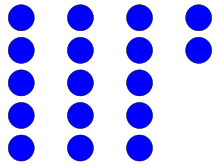## Quotient and Remainder Calculator

 Numerator: Denominator (Divided By) : Quotient : Remainder :

In arithmetic, the division of two integers producing a quotient and a remainder. The number that remains after dividing a number is called as Remainder.

For example, 694÷2 = 347, the quotient is 347. 13÷4, the remainder is 1.17 is divided into 3 groups of 5 with 2 left over. Here the dividend is 17, the divisor is 5, the quotient is 3, and the remainder is 2. 17 = 5 × 3 + 2

Thinkcalculator.com provides you helpful and handy calculator resources.